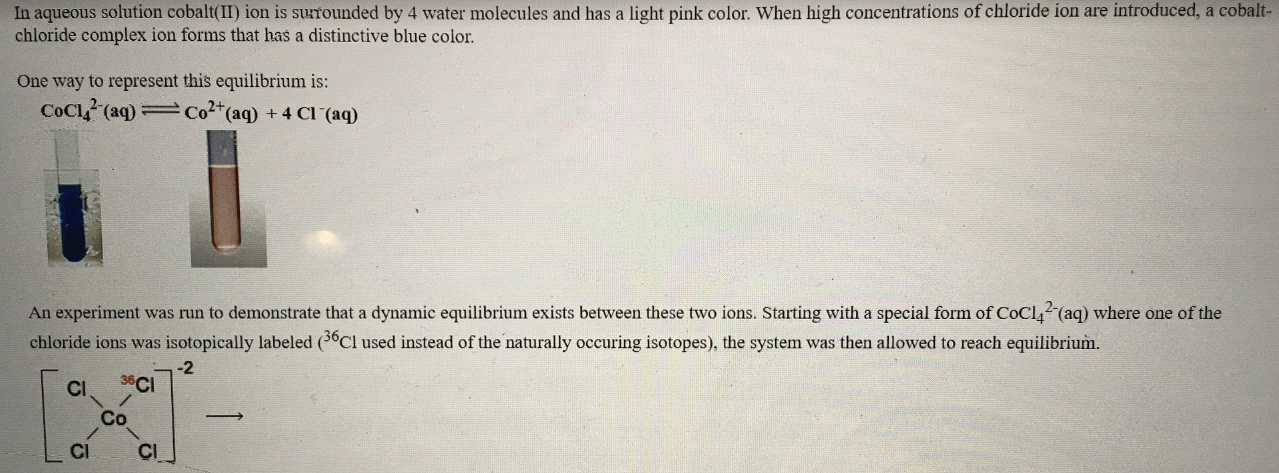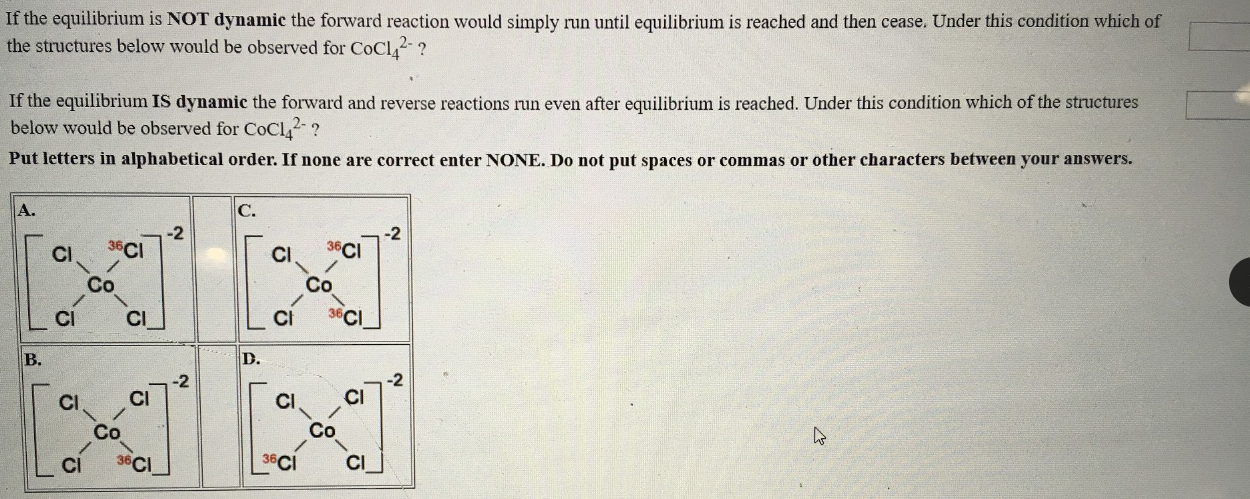# In aqueous solution cobalt(II) ion is surrounded by 4 water molecules and has a light pink color. When high concentrations of chlorine ion are introduced, a cobalt- chloride complex ion forms that has a distinctive blue color. One way to represent this equilibrium is: CoCl4^2-(aq) ⇌ Co^2+(aq) + 4 Cl^-(aq) An experiment was run to demonstrate that a dynamic equilibrium exists between these two ions. Starting with a special form of CoCl4^2-(aq) where one of the chloride ions was isotopically labeled (36Cl used instead of the naturally occuring isotopes), the system was then allowed to reach equilibrium. If the equilibrium is NOT dynamic the forward reaction would simply run until equilibrium is reached and then cease. Under this condition which of the structures below would be observed for CoCl4^2-? If the equilibrium IS dynamic the forward and reverse reactions run even after equilibrium is reached. Under this condition which of the structures below would be observed for CoCl4^2-? Put letters in alphabetical order. If none are correct enter NONE. Do not put spaces or commas or other characters between your answers.